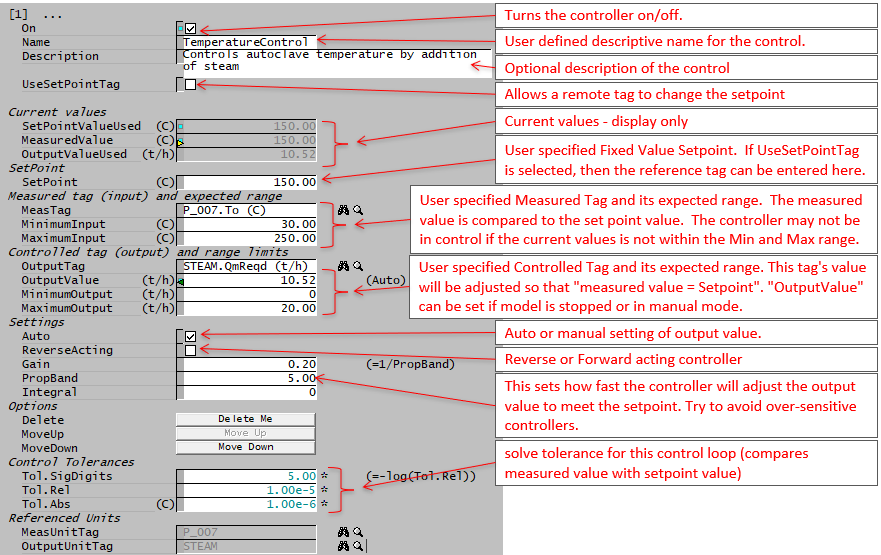# PID Controller

Navigation: Models ➔ Control Models ➔ PID Controller

## General Description

The PID unit is used to simulate simple PID plant control functions. If the user requires more complicated controls that involves data manipulation, then the General Controller can be used to assist the PID control. For example, user may want to calculate the setpoint as a function of temperature, or change the output range as a function of plant feed.

• A single PID controller unit can be used to simulate a number of independent PID controllers on the plant. The user may enter the required number of PID controllers in the Count field on the first page of the Access window.
• The control logic used to manipulate the variables is executed once at the end of each SysCAD iteration.
• The PID controller may be used in Steady State or Dynamic mode.
In Dynamic mode it closely simulates an actual plant PID loop.
In Steady State mode the time dependent factors are not used in the traditional manner, instead each iteration is treated as a "one second time increment".

### The Referenced Tags

The PID Output tag and the Measured tag, will have coloured triangles shown in the access window of those units. When the user right clicks on one of these referenced tags it will indicate which PID is referencing the tag. See Controlled and Referenced Variables.

• In Steady State, the requirement for the measured value to equal the set-point is included in the convergence criteria. Therefore, the SysCAD Steady State solver will continue with iterations until this objective is met within the defined tolerances for at lease three consecutive iterations.
• If the output of a controller is at it's minimum or maximum output limit, then the PID is excluded from these criteria and the solver can stop even though the set-point is not achieved. This is because the output can no longer influence the measured value to achieve the required set-point value. A condition error message is given to alert the user to this.
• Please refer to section Hints and Comments for more comprehensive hints on how to troubleshoots the controller, user can also have a look at the video links below for how to add and troubleshoot the controllers used in the tutorial project.

The following videos are part of the Tutorial showing users how to use PID Controllers:

### Diagram

The diagram shows the default drawing of a PID controller.

This screen dump shows variables used and calculated in the PID Controller. (Example PID from the Tutorial)## Inputs and Outputs

There are no connections to this unit.

## Model Theory

The classical PID algorithm as shown below is used in the model:

$\mathbf {\mathrm{u(t) = K e(t)+\cfrac{1}{T_i}\int e(t)dt + T_d\cfrac{de(t)}{dt}}}$

Where:

u(t) = the control action or output variable at time t in seconds;
e(t) = the error (or deviation) w(t) - y(t): the difference between the set point w(t) and the measured variable y(t);
K = the Gain;
Ti = the Integral time;
Td = the Derivative time.

For a full description of the theory, please see the reference below.